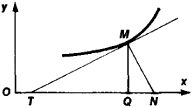# Subtangent and Subnormal

The following article is from The Great Soviet Encyclopedia (1979). It might be outdated or ideologically biased.

## Subtangent and Subnormal

the directed line segments QT and QN that are the projections on the x-axis of the segments MT and MN of the tangent and normal, respectively, to some curve at the point M of the curve (see Figure 1). If the curve is the graph of the function y = f(x), the length of the subtangent QT is equal to — f(x) / fʹ (x), and the length of the subnormal QN is equal to f(x)fʹ(x), where x is the abscissa of M. If the curve is defined parametrically— that is, x = Φ(t), y = ψ(t) — the length of QT is– ψ(t)Φʹ (t)/ψ’(t) and the length of QN is ψ(t)ψʹ (t/Φʹ (t), where t is the parameter that determines the position of M on the curve.Figure 1